### Home > PC > Chapter 2 > Lesson 2.2.1 > Problem2-42

2-42.
1. Expand each of the following sums and find them. Homework Help ✎

1.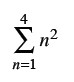2.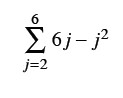3.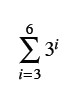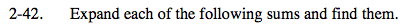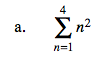12 + 22 + 32 + 42

30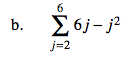(6(2) − 22) + (6(3) − 32) + (6(4) − 42) + (6(5) − 52) + (6(6) − 62)
Now evaluate the sum.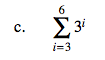Follow the same process as shown in parts (a) and (b).
Be sure to note the starting and ending indices!